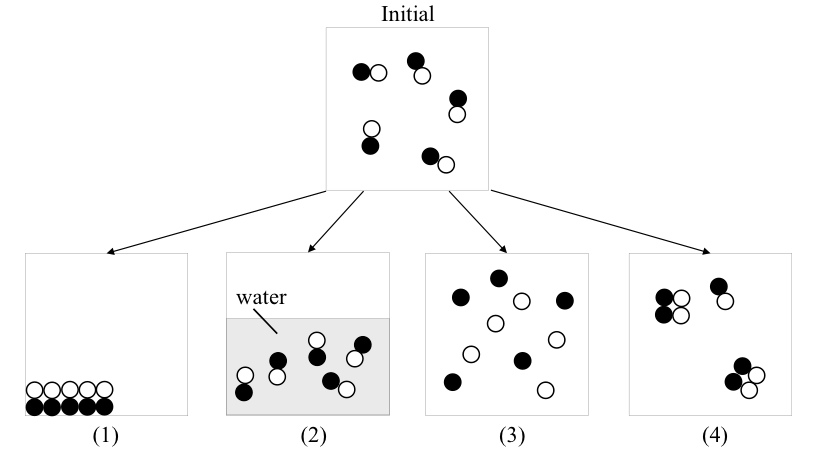# Problem: Consider the following figure of four processes from the initial state. The substances are in gas phase initially. Which process(es) would result in an entropy increase?   A. (1) and (2)  B. (1) and (3) C. only (3) D. (1), (2), and (4) E. only (4)

###### Problem Details

Consider the following figure of four processes from the initial state. The substances are in gas phase initially. Which process(es) would result in an entropy increase?

A. (1) and (2)

B. (1) and (3)

C. only (3)

D. (1), (2), and (4)

E. only (4)